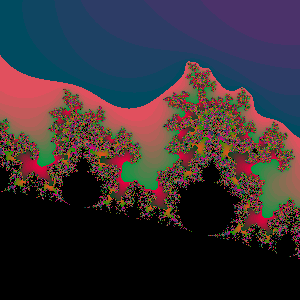## Sunday, June 24, 2007

### Creating fractal images using C# 3.0 features

In this post I'm going to try to implement the basic Escape time algorithm for creating fractal images. My main goal is to use as many C# 3.0 features as possible .

This post is inspired by the very nice blog post A Ray Tracer in C#3.0.

The Escape time algorithm is very simple, but depending on its input it can generate very complex images.

Although not mandatory, the first thing to create is a class from complex numbers. The complex number class contains the following members:

``public class Complex{   public Complex(double real, double img)   public Complex Multiply(Complex c)   public Complex Add(Complex c)   public double Norm()   public double NormSquared()   public static Complex operator *(Complex c1,Complex c2)    public static Complex operator +(Complex c1, Complex c2)   public double Real   public double Img }``

Now we need something to convert from the image coordinate system to the real coordinate system. To do this the following function was created:

``public static Func<double, double> InterpFunc(double x1,double y1,double x2,double y2){   double m = (y2 - y1) / (x2 - x1);   double b = y1 - (m * x1);   return (x => m * x + b);}``

The `InterpFunc` method creates a function that maps values from one coordinate system to the other.

The code that creates the image is the following:

``void CreateFractal(double minX, double minY,double maxX, double maxY,int imageWidth, int imageHeight){   Func<double, double> xF = MathUtils.InterpFunc(0, minX, imageWidth, maxX);   Func<double, double> yF = MathUtils.InterpFunc(0, minY, imageHeight, maxY);   foreach (var p in from yi in Enumerable.Range(0, imageHeight)                     from xi in Enumerable.Range(0, imageWidth)                     select new                       {                          x = xi,                          y = yi,                          xD = xF(xi),                          yD = yF(yi)                       })   {      Complex p0 = new Complex(p.xD, p.yD);      Func<Complex, Complex> function = functionConstructor(p0);      int i = ApplyFunction(function, p0)              .TakeWhile(                (x, j) => j < maxIteration && x.NormSquared() < 4.0)              .Count();      HandlePixel(p.x, p.y, i);   }}``

Basically `select ` expression generates the coordinates for all the points in the image . The `functionConstructor` function is used to create the function that will be applied to generate the fractal. One example of this functions is the one used for the Mandelbrot fractal `f(x) = x^2 + p0` . The escape time is calculated by counting the number of elements in the generated sequence of function applications before the value escaped.

The `ApplyFunction` method is interesting since is the one that creates the sequence of recursive function applications required for this algorithm. The method looks like this:

``IEnumerable<Complex> ApplyFunction(Func<Complex, Complex> function,Complex initial){   Complex last = initial;   while (true)   {      last = function(last);      yield return last;   }}``

By running this algorithm using the Mandelbrot formula:

``Func<Complex,Func<Complex,Complex>> fc =       p0 => (c => c.Multiply(c).Add(p0));EscapeTimeFractal p = new EscapeTimeFractal(300, 300, fc);p.MinX = -0.03;p.MinY = 0.68;p.MaxX = 0.03;p.MaxY = 0.62;p.Run();``

The generated image is the following:By running it using the Szegedi Butterfly 1 formula:

``Func<Complex,Func<Complex,Complex>> fc =       p0 =>        (c => (new Complex(                  ((c.Img * c.Img) - Math.Sqrt(Math.Abs(c.Real))),                  ((c.Real * c.Real) - Math.Sqrt(Math.Abs(c.Img)))))                + p0);EscapeTimeFractal p = new EscapeTimeFractal(300, 300,fc);p.MinX = -2;p.MinY = 2;p.MaxX =2;p.MaxY = -2;p.MaxIteration = 127;p.OutputFileName = @"c:\temp\output.bmp";p.Run();``

The generated image is the following:The code for this experiment can be found here.